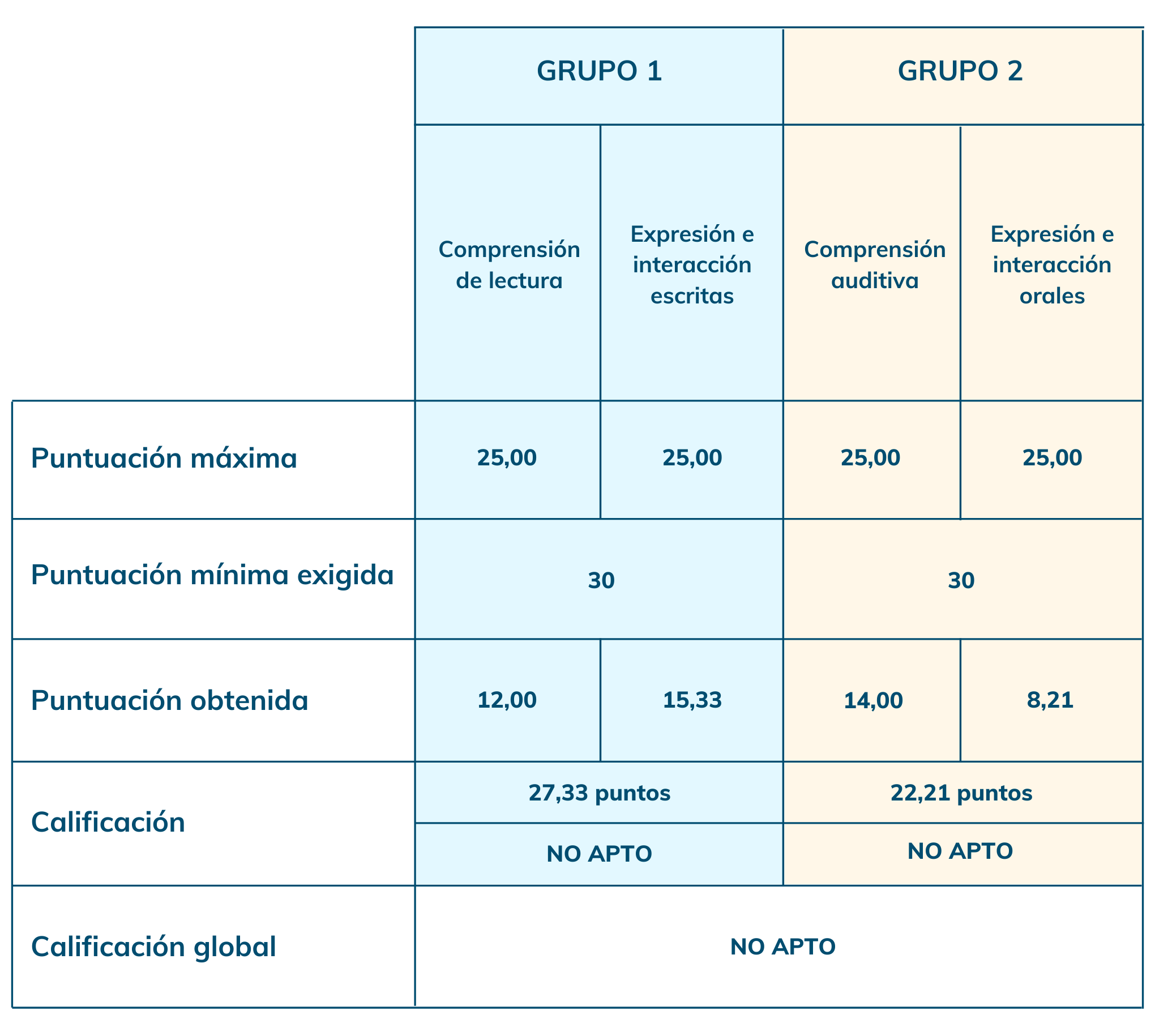# How Is the DELE Scored?

Find out everything about the test scoring!

## Comprehension tests

Only one answer per question is correct. This enables an automatic correction of the answers.

• A correct answer is worth one point.
• The maximum score that can be obtained is therefore equal to the number of questions in the test. Once the score has been calculated, it is converted to a 25-point scale using the following formula:For example, if a candidate gets a grade of 22 out of 30 in the written comprehension part of the test, their grade on a scale of 25 points would be:
(22 x 25) / 30 = 18,3 points

## Expression and interaction tests (writing and speaking)

These sections are graded by trained and accredited examiners who rely on two categories to grade the students:

• o The analytical category is composed of several criteria. Each criterion is scored between 0 and 3 points.
• The holistic category (scored from 0 to 3 points)
• Once the score has been attributed for each criterion and category, an average is calculated to get to a final score between 0 and 3. Then, to convert this score on a scale of 25 points, you can use the following formula:For example, if a candidate gets 1 point at the test of oral expression, their grade, converted on a scale of 25 points, will be the following:
(1 x 25) / 3 = 8,33 points

## Concerning the final score of the DELE

The results are sent by e-mail approximately 3 months after the completion of the written tests. The Cervantes Institute sends an e-mail to the candidates so that they can check their results through the Cervantes Institute online portal.

At the bottom of the certificate, you will find the following information: "APTO" (if you passed the exam) or "NO APTO" (if you failed the exam).

## Requirements for the DELE B1 and B2:

In order to pass the DELE B1 and B2 exams, it is necessary to:

• Obtain a minimum score of 60 points out of 100 for the entire exam.
• Obtain at least 30 points out of 50 in each group of tests.
• Here is an example of scores for the 4 sections:If a candidate can see "APTO" at the bottom of his/her results, a diploma will be sent to his/her postal address provided by the candidate (be sure to keep your postal address up to date with the test center where you took the test).